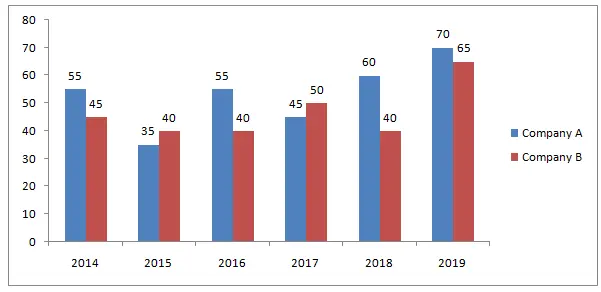# SBI Clerk Prelims 2021 Quantitative Aptitude Questions (Day-27)

Dear Aspirants, Our IBPS Guide team is providing new series of Quantitative Aptitude Questions for SBI Clerk Prelims 2020 so the aspirants can practice it on a daily basis. These questions are framed by our skilled experts after understanding your needs thoroughly. Aspirants can practice these new series questions daily to familiarize with the exact exam pattern and make your preparation effective.

Start Quiz

Bargraph

Directions (01-05): Study the following information carefully and answer the questions given below.

The below graph shows the profit percentage earned by two companies A and B over the given years.

Profit% = (Income – Expenditure)/Expenditure * 1001) The income of two companies A and B in 2018 were in the ratio of 5: 4 respectively. What is the respective ratio of their expenditures in 2018?

A.35: 32

B.32: 35

C.12: 13

D.13: 14

E.None of these

2) If the expenditure of company B in 2014 was Rs.280 Crore, what was its income in 2014?

A.202 Crore

B.304 Crore

C.406 Crore

D.400 Crore

E.None of these

3) If the expenditures of company A and company B in 2015 were equal and the total income of the two companies in 2015 was Rs.540 Crore, what was the total profit of the two company together in 2015?

A.142.27 crore

B.147.27 Crore

C.152.27 Crore

D.155.27 Crore

E.None of these

4) The expenditure of company A in the year 2016 was Rs.300 Crore and the income of company A in 2016 was the same as its expenditure in 2019. Then what was the income of company A in 2019?

A.790.5 Crore

B.820 Crore

C.845.5 Crore

D.960 Crore

E.None of these

5) If the income of two company were equal in 2017, then what was the ratio of expenditure of company A to that of B in 2017?

A.34: 27

B.27: 34

C.30: 29

D.29: 30

E.None of these

Simplification

Directions (06-10): What value should come in the place of (?) in the following questions?

6) (12.5% of 9696) × 40% of 360 ÷ 12 =?

A.13144

B.17854

C.14454

D.14544

E.None of these

7) 0.75% of 1575 – 1.25% of 1000 + 1/11 × 5775 =?

A.524.3125

B.521.7125

C.523.3125

D.529.7125

E.None of these

8) X % of 750 – 1600 + 3015 ÷ 15 × 225 = 44225

A.75

B.80

C.85

D.70

E.None of these

9) 1 7/3 + 33/6 + 7 11/9 – ? = 1 6/9

A.136/9

B.221/18

C.275/18

D.277/18

E.None of these

10) (121)× 847 × 11/7 × 1/11 × 1331 = (11)X

A.13

B.11

C.10

D.12

E.None of these

(160% of E1)/(140% of E2) = 5/4

=> 16/14 * E1/E2 = 5/4

=> E1/E2 = 35/32

Income = Expenditure + P% * Expenditure

= 280 + 45% * 280 = 406 Crore

Expenditure of company A and company B in 2015 be 100.

Then, 100 + 35 + 100 + 40 = 275

Required profit = 540/275 * 75 = 147.27 Crore

Expenditure of company A in 2016 = 300 Crore

Income of company A in 2016 = 300 + 55% of 300

= 300 + 165 = 465 Crore

Expenditure of company A in 2019 = 465 Crore

Income of company A in 2019 = 465 + 70% of 465 = 790.5 Crore

145A/100 = 150B/100 be the incomes of two companies in 2017

A/B = 150/145 = 30/29

(1/8 × 9696) × 40% of 360 ÷ 12 =?

1212 × 144 ÷ 12 =?

1212 × 12 =?

? = 14544

0.75% of 1575 – 1.25% of 1000 + 1/11 × 5775 =?

11.8125 – 12.5 + 525 =?

? = 524.3125

X % of 750 – 1600 + 3015 ÷ 15 × 225 = 44225

X % of 750 – 1600 + 201× 225 = 44225

X % of 750 – 1600 + 45225 = 44225

X % of 750 + 43625 = 44225

X % of 750 = 600

X/100 × 750 = 600

X = 80

1 7/3 + 33/6 +7 11/9 – 1 6/9 =?

10/3 + 33/6 + 74/9 – 15/9 =?

277/18 =?

(121)× 847 × 11/7 × 1/11 × 1331 = (11)X

(112)× 121 × 11 × 1/11 × 1331 = (11)X

(11)× (11)2 × (11)3 = (11)X

(11)11 = (11)X

x = 11

 Check Here to View SBI Clerk Prelims 2021 Quantitative Aptitude Questions Day – 26 Day – 25 Day – 24
5 1 vote
Rating